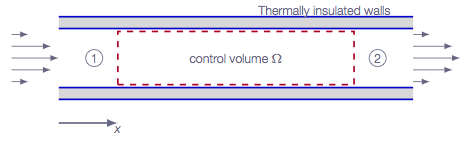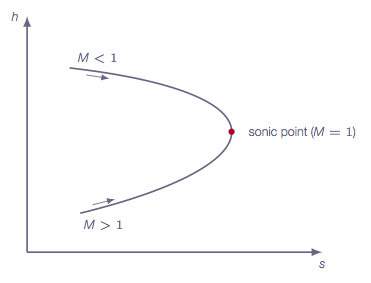## Chapter 3

One-Dimensional Flow

## One-Dimensional Flow

### Overview

The third chapter in the book deals with flows that can be assumed to be one dimensional, i.e. flow quantities will only vary in one direction. Although this may seem to be of very limited use, the theory covered and equations derived in this section will be of great help in the coming chapters. The specific model problems analyzed in detail in chapter three are: normal shocks, one-dimensional flow with heat addition, and one-dimensional flow with friction.

### Online Flow Calculator

Sections of the online flow calculator for compressible flow problems CFLOW related to this chapter:

• Section 1: Gas Relations
• Section 2: Total Flow Properties
• Section 3: Normal Shock Relations
• Section 4: 1D Flow with Heat Addition
• Section 5: 1D Flow with Friction

The CFLOW finite-volume quasi-1D solver can also be used to study the problems in this chapter.

### Sections

#### 3.2 One-dimensional Flow Equations

The one dimensional flow equations are derived starting from the conservation equations on integral form introduced in Chapter 2. With variation of flow quantities in one direction only, a simplified set of governing equations is derived using a control volume approach.

conservation of mass

$$\rho_1 u_1 = \rho_2 u_2$$

conservation of momentum

$$p_1 +\rho_1 u_1^2 = p_2 + \rho_2 u_2^2$$

conservation of energy

$$h_1 +\frac{u_1^2}{2} = h_2 + \frac{u_2^2}{2}$$

#### 3.3 Speed of Sound and Mach Number

Using the above derived equations and choosing a control volume such that it includes the wave front of an acoustic wave the speed of sound is investigated. Using the fact that an acoustic wave is a very weak wave we can make assumptions about changes in flow quantities and losses that leads us to a relation between the speed of sound and the compressibility

$$a^2=\left(\frac{\partial p}{\partial \rho}\right)_s$$

Finally, by assuming that we can treat the gas as calorically perfect, we end up with the following relation between speed of sound and temperature

$$a=\sqrt{\gamma RT}$$

#### 3.4 Some Conveniently Defined Flow Parameters

This section introduces total and characteristic flow properties. The total properties represent the condition that we would have if we could slow the flow down to zero velocity isentropically (without losses). The characteristic properties represent the condition that we would have if we could accelerate or decelerate the flow adiabatically to sonic conditions.

#### 3.5 Alternative Form of the Energy Equation

Using the definition of speed of sound for calorically perfect gases and the relations derived in the previous section one can rewrite the energy equation to obtain ratios of total and local flow properties and ratios of characteristics and local flow properties, respectively.

#### 3.6 Normal Shock Relations

Applying the control volume approach to a one-dimensional flow with a normal shock situated within the control volume leads to the normal shock relations. The normal shock relations are expressions relating ratios of flow quantities from regions upstream of the shock and corresponding properties on the downstream side to the upstream Mach number. The relation between the characteristics Mach number on the upstream side $$M^*_1$$ and the characteristic Mach number on the downstream side of the normal shock $$M^*_2$$ tells us that behind a normal shock the flow is always subsonic.

Analysing the energy equation and the second law of thermodynamics shows that there is a direct relation between entropy increase and total pressure drop.

#### 3.7 The Hugoniot Equation

The Hugoniot equation is an alternative normal shock relation based on thermodynamic quantities only.

#### 3.8 One-Dimensional Flow with Heat Addition

With heat addition in our control volume we end up with a modified energy equation. It is shown that the direct effect of the added heat is an increase of total temperature.

$$q=C_p(T_{o2}-T_{o1})$$

It is shown that adding heat will bring the flow Mach number closer to unity. If the flow is subsonic, adding heat will increase the flow Mach number until the flow reaches sonic conditions. After that adding more heat will not be possible without changing the inflow conditions. For a supersonic flow, adding heat will lower the Mach number until the flow is sonic.

In this section a new set of starred flow quantities are introduced. In this case the star denotes the flow properties that we would get if enough heat was added to reach sonic conditions. The starred quantities are constants in a given flow and can thus be used as a reference state when solving problems.

#### 3.9 One-Dimensional Flow with Friction

The governing equations for one-dimensional flow with friction are derived. The introduction of friction gives results in the addition of an integral in the momentum equation and the equations are transformed to differential form. In the same way as adding heat lead to changed flow properties, the effect of the friction will be that the Mach number of a subsonic flow will be increased with increased pipe length until sonic conditions are reached and the Mach number of a supersonic flow will be decreased.Figure 6: Investigating one-dimensional flow with friction using a control volume approach

A new set of starred quantities are introduced. For one-dimensional flow with friction, the starred flow properties represent the condition that we would have if the pipe was long enough to reach sonic conditions at the end. When sonic conditions are reached $$L=L^*$$, the pipe cannot be made longer without modifying the inlet boundary conditions.Figure 7: Fanno curve - Mollier diagram for one-dimensional flow with friction

### Study Guide

The questions below are intended as a "study guide" and may be helpful when reading the text book.

#### Auxiliary Relations

1. How is the speed of sound defined, for any type of gas?
2. Derive an expression for the speed of sound for a calorically perfect gas $$a=\sqrt{\gamma R T}$$ starting from the general formula: $$a^2=\left(\frac{\partial p}{\partial \rho}\right)_s$$
3. What is the definition of Mach number?
4. How do we define total conditions in a steady-state isentropic flow?
5. How do we define critical conditions in a steady-state isentropic flow?
6. For a steady-state isentropic flow of a calorically perfect gas, derive the formula for $$T_o/T$$, making use of the fact that the total enthalpy $$h_o$$ is constant along the streamlines. $$\dfrac{T_0}{T}=1+\dfrac{1}{2}(\gamma-1)M^2$$
7. How is the transformed Mach number $$M^*$$ defined?
8. How is $$M^*$$ related to the flow Mach number $$M$$?
9. For a steady-state adiabatic compressible flow of calorically perfect gas, which of the variables $$p_0$$ (total pressure) and $$T_0$$ (total temperature) is/are constant along streamlines? Why?
10. What is the general definition (valid for any gas) of the total conditions $$p_0$$, $$T_0$$, $$\rho_0$$,... etc at some location in the flow?
11. In Formulas, Tables & Graphs, check formulas on pages (5-6). You should recognize the formulas except for the chemically reacting mixture... case.

#### Stationary Normal Shock in One-Dimensional Flow

1. How do we apply the control volume approach, for conservation of mass, momentum and energy, to this case in order to derive the three main equations?
2. How many additional relations are needed to solve for the conditions downstream of the shock, given the upstream conditions?
3. Is it possible to obtain an analytic solution to the shock problem for any type of gas?
4. The equation actually allow two solutions, one with an entropy increase across the shock (compression shock) and one with an entropy decrease across the shock (expansion shock). What thermodynamic principle guides us in the choice of the physically correct solution, and which solution is the correct one?
5. Describe how different variables (e.g. pressure, density, temperature, velocity, Mach number, total pressure, total temperature, entropy) change across a stationary normal shock, when going from the upstream side to the downstream side (following the flow).
6. Assume a steady-state 1D flow with a stationary normal shock. The fluid particles crossing the shock are subjected to
1. a pressure drop
2. a density increase
3. an entropy increase
4. a temperature drop
5. a deceleration
Which statements are true and which are false?
7. Are the normal-shock relations mathematically and physically valid for upstream Mach numbers lower than one? Justify your answer.
8. How come that the control volume approach applied to the governing equations for adiabatic form gives us the normal-shock relations? i.e., how do the equations "know" that there is a shock inside of the control volume?
9. Explain in words what the Hugoniot equation is. How does this equation differ from the other normal-shock relations derived in Chapter 3?
10. In Formulas, Tables & Graphs, check formulas on page (7). You should recognize the formulas and be familiar with the notation.

#### One-Dimensional Flow with Heat Addition

1. How do we apply the control volume approach, for conservation of mass, momentum and energy, to this case in order to derive the three main equations?
2. Is it possible to obtain an analytic solution for any type of gas?
3. Derive the relation between the change in total temperature between point 1 and point 2 and the added heat per unit mass.
4. What is the Rayleigh curve and what does it tell us?
5. The general solution for calorically perfect gas is given in terms of conditions at point 1 and point 2. Describe how these formulas can be simplified by introducing the concept of sonic point conditions.
6. Describe how different variables (e.g. pressure, density, velocity, Mach number, total pressure, total temperature, entropy) change from point 1 to point 2 when heat is added.
7. How could heat addition theoretically be used to generate a supersonic flow?
8. Looking at the Rayleigh curve it's evident that removing heat leads to reduced entropy - how come that this is possible?
9. In Formulas, Tables & Graphs, check formulas on page (8-9). You should recognize the formulas and be familiar with the notation.

#### One-Dimensional Flow with Friction

1. How do we apply the control volume approach, for conservation of mass, momentum and energy, to this case in order to derive the three main equations?
2. Is it possible to obtain an analytic solution for any type of gas?
3. What is the Fanno curve and what does it tell us?
4. The general solution for calorically perfect gas is given in terms of conditions at point 1 and point 2. Describe how these formulas can be simplified by introducing the concept of sonic point conditions.
5. Describe how different variables e.g. pressure, density, velocity, Mach number, total pressure, temperature, entropy) change from point 1 to point 2 due to friction.
6. Does the total temperature $$T_o$$ change due to friction?
7. Describe the choking of flow that occurs for pipe flow with friction. What happens if the real length $$L$$ of a pipe is longer than $$L^*$$ (for either subsonic flow or supersonic flow)?
8. In Formulas, Tables & Graphs, check formulas on page (10-11). You should recognize the formulas and be familiar with the notation.